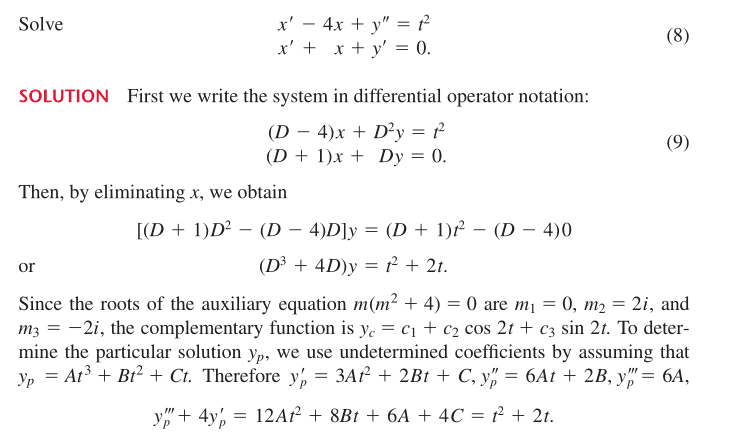# A question on undetermined coefficients

## Homework Statement

http://gyazo.com/6c440aa92106f729639c91f6d59dcd89

## The Attempt at a Solution

My question is why is yp = At^3+Bt^2 +Ct. The reason I ask that is because is see t^2+2t so why wouldnt it be yp=At^2 +Bt +c?

SammyS
Staff Emeritus
Science Advisor
Homework Helper
Gold Member

## Homework Statement

http://gyazo.com/6c440aa92106f729639c91f6d59dcd89

## The Attempt at a Solution

My question is why is yp = At^3+Bt^2 +Ct. The reason I ask that is because is see t^2+2t so why wouldn't it be yp=At^2 +Bt +c?Because (D3 + 4D)( At3+Bt2 +Ct) = 12At2 + 8Bt + 6A + 4C .

Whereas, (D3 + 4D)( At2+Bt+C) = 8At + 4B, which is not a quadratic.

.